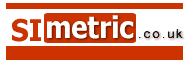###### I will be honest with you. There is no easy way to learn your times tables. You will have to try hard to memorise them 'parrot fashion'. Believe me, it is the only way.Read the tables again and again and again to commit them to memory for ever. The Times Tables.
 [ home ] The Times Tables are one of the foundation stones of your education. Like being able to count, write and spell, it is important to be able to multiply. You will need it in every subject and later at work and play. I will be honest with you. There is no easy way to remember your tables. You will just have to accept the fact that you will have to read them over and over again till you remember them so deeply that the answers just pop out without trying. Just like you remember your name. After you have done the hard work and learnt your times tables you can have some fun testing yourself, but first .. Print this page and read each table aloud 10 times a day. If you can understand that sums like 7 x 6 are the same as 6 x 7 then you only have to learn about half as much. Learn off by heart the sums in white at the bottom and see where they are repeated in grey above.

 2 x table 3 x table 4 x table 5 x table 6 x table 7 x table 8 x table 9 x table 1 2's are 2 1 3's are 3 1 4's are 4 1 5's are 5 1 6's are 6 1 7's are 7 1 8's are 8 1 9's are 9 2 2's are 4 2 3's are 6 2 4's are 8 2 5's are 10 2 6's are 12 2 7's are 14 2 8's are 16 2 9's are 18 3 2's are 6 3 3's are 9 3 4's are 12 3 5's are 15 3 6's are 18 3 7's are 21 3 8's are 24 3 9's are 27 4 2's are 8 4 3's are 12 4 4's are 16 4 5's are 20 4 6's are 24 4 7's are 28 4 8's are 32 4 9's are 36 5 2's are 10 5 3's are 15 5 4's are 20 5 5's are 25 5 6's are 30 5 7's are 35 5 8's are 40 5 9's are 45 6 2's are 12 6 3's are 18 6 4's are 24 6 5's are 30 6 6's are 36 6 7's are 42 6 8's are 48 6 9's are 54 7 2's are 14 7 3's are 21 7 4's are 28 7 5's are 35 7 6's are 42 7 7's are 49 7 8's are 56 7 9's are 63 8 2's are 16 8 3's are 24 8 4's are 32 8 5's are 40 8 6's are 48 8 7's are 56 8 8's are 64 8 9's are 72 9 2's are 18 9 3's are 27 9 4's are 36 9 5's are 45 9 6's are 54 9 7's are 63 9 8's are 72 9 9's are 81 10 2's are 20 10 3's are 30 10 4's are 40 10 5's are 50 10 6's are 60 10 7's are 70 10 8's are 80 10 9's are 90
 Tips. Naught times table not shown. It is too easy. 0 times any number is still 0. Imagine three bits of nothing - you still have nothing. The 10 times table is also not shown because it is too easy. 10 x 10 = 100 I learned the 11 times, 12 times and 16 times tables at school to help with inches and ounces - so can you! Any number times 1 remains the same number. See the blue row. Also, any number divided by 1 remains the same number. For example, 8 divided by 1 equals 8. For 1 2's are 2 say aloud 'one twos are two'. For 5 2's are 10 say aloud 'five twos are ten' and so on. In later life, this is how you will be remembering the tables so you might as well learn it from the start. The word 'times' is usually replaced with a little cross 'x' . It is quicker to write 1 x 6 than 1 times 6 It is easier to practice with a friend. Read the tables together with rhythm, like in a song. Then one can say the first part 'one fives are' and the other friend can answer 'five'